### Falling Balls: golf ball versus ping pong ball • Name:

We will be calculating average velocities and average accelerations in order to explore the question of whether the golf ball and ping pong ball fell at different speeds (velocities) and accelerations. In this lab the words speed and velocity will have the same meaning.

1. Open the spreadsheet software Excel and enter both the golf ball the ping pong ball data from your data sheet.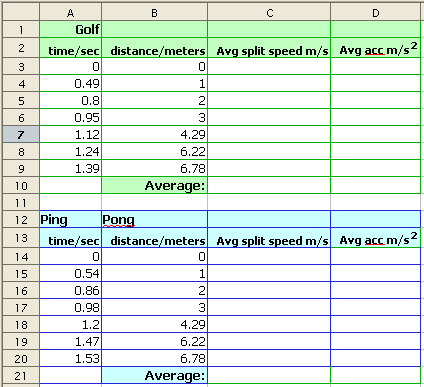2. Start calculating the average speed for each drop height using the formula seen below. Note that the formula will only work if your spreadsheet is set up identically. The formula divides the distance in column B by the time to fall in column A. The result is a speed in meters per second.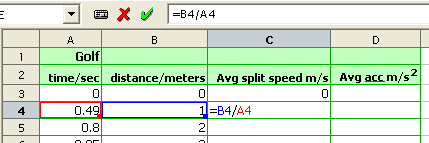3. Fill the formula down by dragging the fill control in the lower right corner of cell C4. Fill down to C9.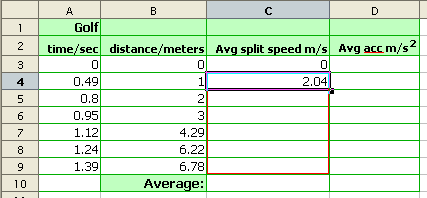4. In C10 use the AVERAGE function to calculate the overall average of the drop velocities. Note that the formula starts with an equals sign.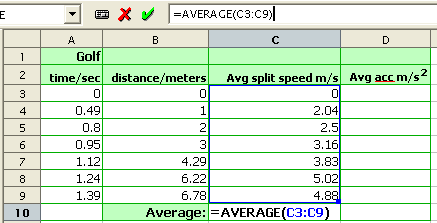5. In D4 calculate the average acceleration.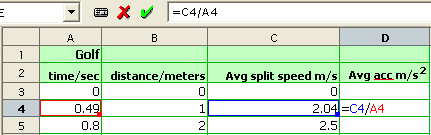6. Fill the average acceleration formula down to D9. In the image below the formula is not yet complete. The image shows the layout during the mouse drag to enter the range. The full formula is =AVERAGE(D4:D9)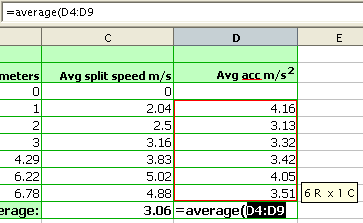7. Now do the same calculations for the ping pong ball. Start with the average velocity.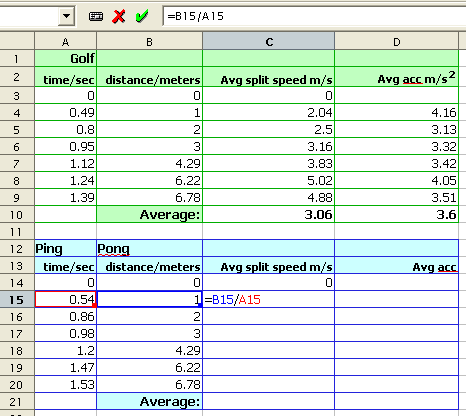8. Fill down and then use the AVERAGE function to calculate the overall average drop velocity for the ping pong ball for each height.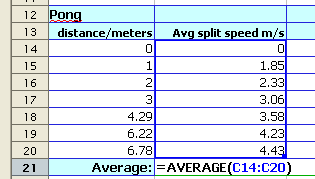9. Next calculate the average acceleration for each drop height.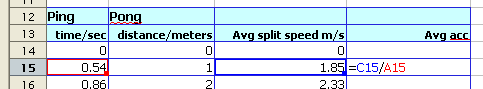10. Fill the average acceleration formula down and calculate the overall average acceleration for the ping pong ball.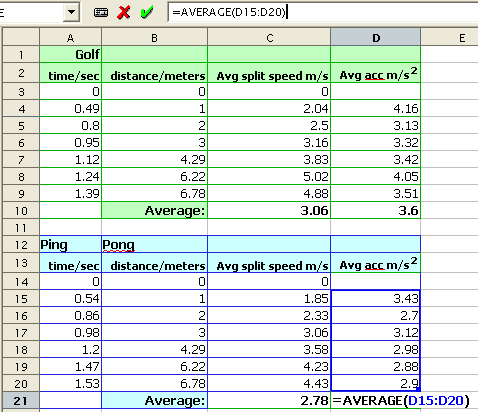11. Which ball has the higher overall average velocity?
12. Why?
13. Which ball has the higher acceleration?
14. Why?
15. The formula velocity = acceleration × time governs the relationship between velocity, acceleration and time. Use the golf ball overall average velocity and the golf ball overall acceleration to solve for the overall average fall time.
Formula: v = at
Solve for t: 3.06 = 3.6t
16. We can use the above formula to calculate the average golf ball velocity after a drop of ten seconds:
v = 3.6(10) = 36 m/s
Find the average golf ball velocity after twenty seconds.
17. The formula distance = ½ × acceleration × time² governs the relationship between distance, acceleration and time. Use the golf ball overall average fall time calculated above and the golf ball overall acceleration to calculate the average golf ball fall distance.
Formula: d = ½at²
Calculate d: d = ½(3.6)(overall average time from above)²

Homework

18. The formula velocity = acceleration × time governs the relationship between velocity, acceleration and time. Use the ping pong ball overall average velocity and the ping pong ball overall acceleration to solve for the overall average fall time.
Formula: v = at
Solve for t: 3.00 = 2.78t
19. We can use the above formula to calculate the average ping pong ball velocity after a drop of ten seconds:
v = 3.00(10) = 300 m/s
Find the average ping pong ball velocity after twenty seconds.
20. The formula distance = ½ × acceleration × time² governs the relationship between distance, acceleration and time. Use the ping pong ball overall average fall time calculated above and the ping pong ball overall acceleration to calculate the average ping pong ball fall distance.
Formula: d = ½at²
Calculate d: d = ½(3.00)(overall average time from above)²